Print Rate 0 stars Common Core
Lesson size:
Message preview:
Someone you know has shared lesson with you:

To play this lesson, click on the link below:

Hope you have a good experience with this site and recommend to your friends too.

Login to rate activities and track progress.
Login to rate activities and track progress.

A quadrilateral is a four- sided polygon with four angles.

• Four sides.
• Four vertices
• The interior add upto 360 degrees.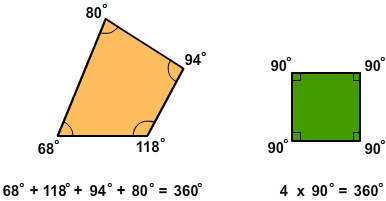The five most common types of quadrilaterals are: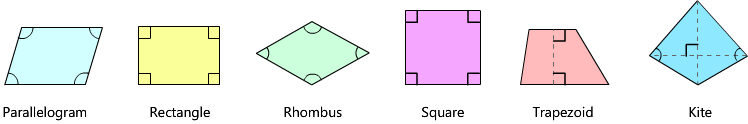## Rectangle

A quadrilateral with four 90-degree angles. Also opposite sides are parallel and of equal length.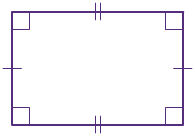## Parallelogram

A quadrilateral with opposite sides parallel. Also opposite angles are equal.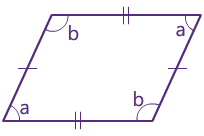## Square

A quadrilateral with four equal sides and four 90 degree angles. Also opposite sides are parallel.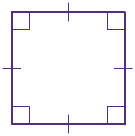## Rhombus

A parallelogram with four equal sides. Also opposite sides are parallel and opposite angles are equal.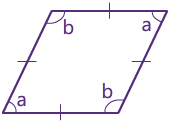## Trapezoid

A quadrilateral that has exactly two sides parallel.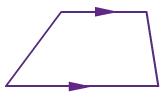## Kite

It has two pairs of sides. Each pair is made up of adjacent sides that are equal in length. The angles are equal where the pairs meet.• A quadrilateral is a four- sided polygon with four angles.
• Four sides.
• Four vertices
• The interior add upto 360 degrees.
• The five most common types of quadrilaterals are:
• Rectangle: A quadrilateral with four 90-degree angles. Also opposite sides are parallel and of equal length.
• Parallelogram: A quadrilateral with opposite sides parallel. also opposite angles are equal.
• Square: A quadrilateral with four equal sides and four 90 degree angles. Also opposite sides are parallel.
• Rhombus: A parallelogram with four equal sides. Also opposite sides are parallel and opposite angles are equal.
• Trapezoid: A quadrilateral that has exactly two sides parallel.
• Kite: It has two pairs of sides. Each pair is made up of adjacent sides that are equal in length. The angles are equal where the pairs meet.

## Similar Lessons

Become premium member to get unlimited access.